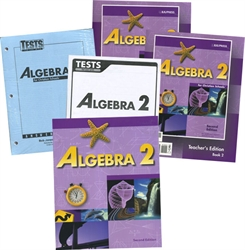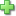BJU Algebra 2 - Home School Kit (old)

2nd Edition, ©2000, Publisher Catalog #201103
Curriculum Bundle
Current Retail Price: \$16.11
Not in stock

Scope & Sequence

• Operations: real numbers; polynomial operations; factoring
• Linear equations: solving equations and inequalities; absolute value equations and inequalities; distances on number lines; word problems; compound inequalities
• Linear relations: line graphs; functions; slopes; special functions; operations on functions; linear inequalities; distances and midpoints
• Polynomial relations: quadratic functions; translations; problem solving; quadratic inequalities; zeros of a function; remainder and factor theorems; graphing polynomial functions
• Systems: graphing; substitution; addition method; problem solving; systems of inequalities; linear programming; systems of three variables
• Radical and exponential functions: simplification; operations; equations; functions
• Complex numbers: operations; solutions to quadratic equations; graphs; vectors
• Rational expressions: simplifying; operations; functions; equations; variations; work problems
• Trigonometry: right triangle trigonometry; problem solving; special triangles; radians; functions and graphs; amplitude and period
• Identities: Law of Sines; Law of Cosines; problem solving; proving identities; trigonometric equations
• Inverse functions: inverse trigonometric functions; logarithms; problem solving
• Probability and statistics: permutations; combinations; binomial theorem; laws of probability; descriptive statistics; standard normal distribution
• Analytical geometry: circles; parabolas; ellipses; hyperbolas; systems of Quadratic Relations
(Each chapter begins with an application from real data correlated to that chapter. Sports, health, nutrition, politics, highway safety, and astronomy provide some of the data sources. Throughout the chapter, statistical analyses will optionally be applied to the data with mathematical technology. Each chapter also has a feature on matrix algebra.)

Includes:Click here to write a review